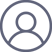李兴旺2018-1-24 00:25

1. 正交  假设x和y为两个随机变量(随机过程同样适用，只需要考虑两个维度)

随机变量：定义R(x, y) = E[xy]为相关函数：若R=0，称x、y正交(注意，相关函数为0，是正交，不是不相关)。

随机过程：定义E[X(t)Y(t)] = 0，则X(t)、Y(t)相互正交

2. 不相关

随机变量：定义 E[xy] = E[x]E[y]，则X、Y不相关。

随机过程：定义E[X(t)Y(t)] = E[X(t)]E[Y(t)]，则X(t)、Y(t)相互不相关

注：不相关和相互独立一般等价，只有当过程为高斯过程时才能力

3. 独立：独立一般用他们的概率密度函数来表示。联合分布等于他们各自的独立分布的乘积，则称为独立： p(xy) = p(x)p(y)

4. 协方差的相关和独立: Cov(x, y) = E[(x - M(x))(y = M(y))]为协方差函数，其中M(x)为x的均值。若Cov = 0，则称之为不相关；不相关只是说二者没有线性关系，但是不代表有任何关系。

独立，不相关 (充分条件)： 独立 -> 不相关

高斯随机变量，独立和不相关充要条件： 独立 <-> 不相关

均值为，不相关和正交充要条件：不相关 <-> 正交

当其中一个期望为零时，“不相关”和“正交”等价，否则没关系。

在通信系统中，总是力图按不相关或正交关系来设计在同一信道随机发送的二元或多元信号。对于多数通信信号以及噪声来说，基本上均值都为0，于是在实际应用中，不相关与正交没有本质区别。